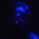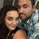2907 vizualizações
Moving Average Crossover Indicator

Here I present a moving average indicator with 9 user definable moving averages from which up to 5 pairs can be selected to show what prices would need to be closed at on the current bar to cross each individual pair.

I have put much emphasis here on simplicity of setting the parameters of the moving averages, selecting the crossover pairs and on the clarity of the displayed information in the optional “Moving Average Crossover Level” Information Box.

What Is a Moving Average (MA)?
According to Investopedia - “In statistics, a moving average is a calculation used to analyze data points by creating a series of averages of different subsets of the full data set.
In finance, a moving average (MA) is a stock indicator that is commonly used in technical analysis . The reason for calculating the moving average of a stock is to help smooth out the price data by creating a constantly updated average price.
By calculating the moving average, the impacts of random, short-term fluctuations on the price of a stock over a specified time-frame are mitigated.”

The user can set the color, type ( SMA / EMA ) and length of each of the 9 moving averages.
Then the user may choose 5 pairs of moving averages from the set of 9.

The script will then calculate the price needed to be crossed by the close of the current bar in order to crossover each of the user defined pairs and outputs the results as optional lineplots and/or an Infobox which shows the relevant information in a very clear way.

The user may switch the moving averages, crossover lineplots and infobox on and off easily with one click boxes in the settings menu.

The number of decimal places shown in the Infobox can be altered in the settings menu.

If the price required to cross a pair of moving averages is zero or less, the crossover level will display “Impossible” and the plots will plot at zero. (this helps ameliorate chart auto-focus issues)

Quoting a variety of online resources …….

Understanding Moving Averages (MA)
Moving averages are a simple, technical analysis tool. Moving averages are usually calculated to identify the trend direction of a stock or to determine its support and resistance levels. It is a trend-following—or lagging—indicator because it is based on past prices.

The longer the time period for the moving average, the greater the lag. So, a 200-day moving average will have a much greater degree of lag than a 20-day MA because it contains prices for the past 200 days. The 50-day and 200-day moving average figures for stocks are widely followed by investors and traders and are considered to be important trading signals.

Moving averages are a totally customizable indicator, which means that an investor can freely choose whatever time frame they want when calculating an average. The most common time periods used in moving averages are 15, 20, 30, 50, 100, and 200 days. The shorter the time span used to create the average, the more sensitive it will be to price changes. The longer the time span, the less sensitive the average will be.

Investors may choose different time periods of varying lengths to calculate moving averages based on their trading objectives. Shorter moving averages are typically used for short-term trading, while longer-term moving averages are more suited for long-term investors.

There is no correct time frame to use when setting up your moving averages. The best way to figure out which one works best for you is to experiment with a number of different time periods until you find one that fits your strategy.

Predicting trends in the stock market is no simple process. While it is impossible to predict the future movement of a specific stock, using technical analysis and research can help you make better predictions.

A rising moving average indicates that the security is in an uptrend, while a declining moving average indicates that it is in a downtrend. Similarly, upward momentum is confirmed with a bullish crossover, which occurs when a short-term moving average crosses above a longer-term moving average. Conversely, downward momentum is confirmed with a bearish crossover, which occurs when a short-term moving average crosses below a longer-term moving average.

Types of Moving Averages

Simple Moving Average ( SMA )
The simplest form of a moving average, known as a simple moving average ( SMA ), is calculated by taking the arithmetic mean of a given set of values. In other words, a set of numbers–or prices in the case of financial instruments–are added together and then divided by the number of prices in the set.

Exponential Moving Average ( EMA )
The exponential moving average is a type of moving average that gives more weight to recent prices in an attempt to make it more responsive to new information.
To calculate an EMA , you must first compute the simple moving average ( SMA ) over a particular time period. Next, you must calculate the multiplier for weighting the EMA (referred to as the "smoothing factor"), which typically follows the formula: 2/(selected time period + 1). So, for a 20-day moving average, the multiplier would be 2/(20+1)= 0.0952. Then you use the smoothing factor combined with the previous EMA to arrive at the current value.
The EMA thus gives a higher weighting to recent prices, while the SMA assigns equal weighting to all values.
Script de código aberto

No verdadeiro espírito TradingView, o autor deste script o publicou com código aberto, para que os traders possam compreendê-lo e verificá-lo. Um brinde ao autor! Você pode usá-lo gratuitamente, mas a reutilização deste código em uma publicação é regida pelas Regras da Casa. Você pode favoritá-lo para utilizá-lo em um gráfico.

Quer usar esse script no gráfico?
You may contact me through Krowns Crypto Cave Discord https://discord.gg/
Where I am a moderator, my username there is The Caretaker also.
My Private indicators are available through https://app.krowntrading.net/

## ComentáriosCRYPTO4202
@CRYPTO4202, I did consider that and it would certainly be possible, however as there are 2 moving averages in a pair, which one should it be the same color as? that left me thinking that its better to leave that up to the user to define themselves.... I have made it as simple as I possibly could to change the various settings and configurations..

***Edit.. I misread your post.. as meaning that the crossover plots being automatically colored as one of the pair colors....
In answer to your actual question...... it is not possible to have different colored text within the text box.. its one color for text and one color for background
RespostaCRYPTO4202
@CRYPTO4202, I should add that you are absolutely right,
It would be amazing and should Tradingview ever add that functionality to Pinescript I will most certainly rewrite this script to incorporate that into it.
RespostaCRYPTO4202
@CRYPTO4202, Did you have a dream about the price Bitcoin tops out at on this run by any chance? :) :p
RespostaThanks for all you do Caretaker! I was wondering if it would be possible for me to add 2 more moving averages to this indicator?
Resposta